Please wait a minute...
 Chin. Phys. B, 2020, Vol. 29(11): 110307    DOI: 10.1088/1674-1056/aba614
 GENERAL Prev   Next

# Protecting the entanglement of two-qubit over quantum channels with memory via weak measurement and quantum measurement reversal

Mei-Jiao Wang(王美姣)1, Yun-Jie Xia(夏云杰)2,3, Yang Yang(杨阳)1, Liao-Zhen Cao(曹连振)1, Qin-Wei Zhang(张钦伟)1, Ying-De Li(李英德)1, and Jia-Qiang Zhao(赵加强)1, †
1 Department of Physics and Optoelectronic Engineering, Weifang University, Weifang 261061, China
2 College of Physics and Engineering, Qufu Normal University, Qufu 273165, China
3 Shandong Provincial Key Laboratory of Laser Polarization and Information Technology, Qufu Normal University, Qufu 273165, China
 Abstract  Based on the quantum technique of the weak measurement and quantum measurement reversal (WMR), we propose a scheme to protect entanglement for an entangled two-qubit pure state from four typical quantum noise channels with memory, i.e., the amplitude damping channel, the phase damping channel, the bit flip channel, and the depolarizing channel. For a given initial state | ψ 〉 = a| 00 〉 + d| 11 〉, it is found that the WMR operation indeed helps to protect entanglement from the above four quantum channels with memory, and the protection effect of WMR scheme is better when the coefficient a is small. For the other initial state | ϕ 〉 = b| 01 〉 + c| 10 〉, the effect of the protection scheme is the same regardless of the coefficient b and the WMR operation can protect entanglement in the amplitude damping channel with memory. Moreover, the protection of entanglement in quantum noise channels without memory in contrast to the results of the channels with memory is more effective. For | ψ 〉 or | ϕ 〉, we also find that the memory parameters play a significant role in the suppression of entanglement sudden death and the initial entanglement can be drastically amplified. Another more important result is that the relationship between the concurrence, the memory parameter, the weak measurement strength, and quantum measurement reversal strength is found through calculation and discussion. It provides a strong basis for the system to maintain maximum entanglement in the nosie channel. Keywords:  quantum entanglement      weak measurement and quantum measurement reversal      quantum channel with memory      concurrence Received:  08 May 2020      Revised:  06 July 2020      Accepted manuscript online:  15 July 2020 Fund: the Natural Science Foundation of Shandong Province, China (Grant No. ZR2017MF040). Corresponding Authors:  †Corresponding author. E-mail: zhaojiaqiang@wfu.edu.cn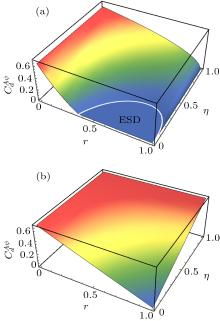Fig. 1.  In the AD channel with memory, the concurrence ${C}_{d}^{A\psi (\phi)}$ is the function of decoherent strength r and the memory parameter η for two initial entangled states | ψ 〉 = a|00〉 + d| 11 〉 and | ϕ 〉 = b| 01 〉 + c| 10 〉. We assume (a) | a | = 1 / 3, (b) | b | = 1 / 3.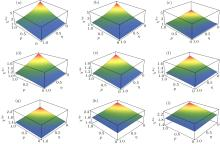Fig. 2.  In the AD channel with memory, χAψ is a function of the weak measurement strength p and the memory parameter η for the initially entangled states | ψ 〉 = a| 00 〉 + d| 11 〉. We assume r = 0.2, (a) $a=\sqrt{0.1}$; (b) a = 1 / 3; (c) $a=\sqrt{0.2}$; (d) $a=\sqrt{0.4}$; (e) $a=\sqrt{2}/2$; (f) $a=\sqrt{0.6}$; (g) $a=\sqrt{0.8}$; (h) $a=\sqrt{8}/3$; (i) $a=\sqrt{0.9}$.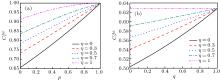Fig. 3.  In the AD channel with memory, the concurrence ${C}_{r}^{A\psi (\phi)}$ is a function of WM strength p (q) for the two initially entangled states | ψ 〉 = a| 00 〉 + d| 11 〉 and | ϕ 〉 = b| 01 〉 + c| 10 〉. We assume r = 0.2, (a) | a | = 1 / 3, (b) | b | = 1/3.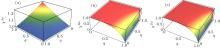Fig. 4.  In the quantum channels with memory, χA(B,D)ϕ is a function of the weak measurement strength p and the memory parameter η for the initially entangled state | ϕ 〉 = b| 01 〉 + c| 10 〉. We assume r = 0.2 and | b | = 1 / 3.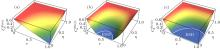Fig. 5.  In the quantum channels with memory, the concurrence ${C}_{d}^{P(B,D)\psi (\phi)}$ is a function of decoherent strength r and the memory parameter η for the two initial entangled states | ψ 〉 = a| 00 〉 + d| 11 〉 and | ϕ 〉 = b| 01 〉 + c| 10 〉. We assume | a | = 1 / 3 and | b | = 1 / 3. (a) PD channel; (b) BF channel; (c) depolarizing channel.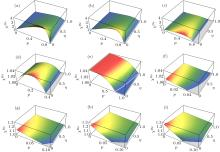Fig. 6.  In the BF channel with memory, ${\chi }^{B\psi }$ is a function of the weak measurement strength p and the memory parameter η for the initially entangled state | ψ 〉 = a| 00 〉 + d| 11 〉. We assume r = 0.2, (a) $a=\sqrt{0.1}$; (b) a = 1 / 3; (c) $a=\sqrt{0.2}$; (d) $a=\sqrt{0.4}$; (e) $a=\sqrt{2}/2$; (f) $a=\sqrt{0.6}$; (g) $a=\sqrt{0.8}$; (h) $a=\sqrt{8}/3$; (i) $a=\sqrt{0.9}$.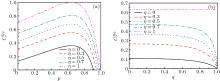Fig. 7.  In the BF channel with memory, the concurrence ${C}_{r}^{B\psi (\phi)}$ is a function of weak measurement strength p (q) for two initially entangled states | ψ 〉 = a| 00 〉 + d| 11 〉 and | ϕ 〉 = b| 01 〉 + c| 10 〉. We assume r = 0.2, (a) | a | = 1 / 3, (b) | b | = 1 / 3.